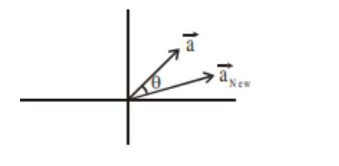# A vector a has components 3p and 1Question:

A vector a has components $3 \mathrm{p}$ and 1 with respect to a rectangular cartesian system. This system is rotated through a certain angle about the origin in the counter clockwise sense. If, with respect to new system, a has components $p+1$ and $\sqrt{10}$, then a value of $p$ is equal to:

1. 1

2. $-\frac{5}{4}$

3. $\frac{4}{5}$

4. $-1$

Correct Option: , 4

Solution:

$\overrightarrow{\mathrm{a}}$ old $=3 \mathrm{p} \hat{\mathrm{i}}+\hat{\mathrm{j}}$

$\overrightarrow{\mathrm{a}}_{\mathrm{New}}=(\mathrm{p}+1) \hat{\mathrm{i}}+\sqrt{10} \hat{\mathrm{j}}$

$\Rightarrow\left|\overrightarrow{\mathrm{a}}_{\mathrm{old}}\right|=\left|\overrightarrow{\mathrm{a}}_{\mathrm{New}}\right|$

$\Rightarrow \mathrm{ap}^{2}+1=\mathrm{p}^{2}+2 \mathrm{p}+1+10$

$8 \mathrm{p}^{2}-2 \mathrm{p}-10=0$

$4 p^{2}-p-5=0$

$(4 p-5)(p+1)=0 \rightarrow p=\frac{5}{4},-1$